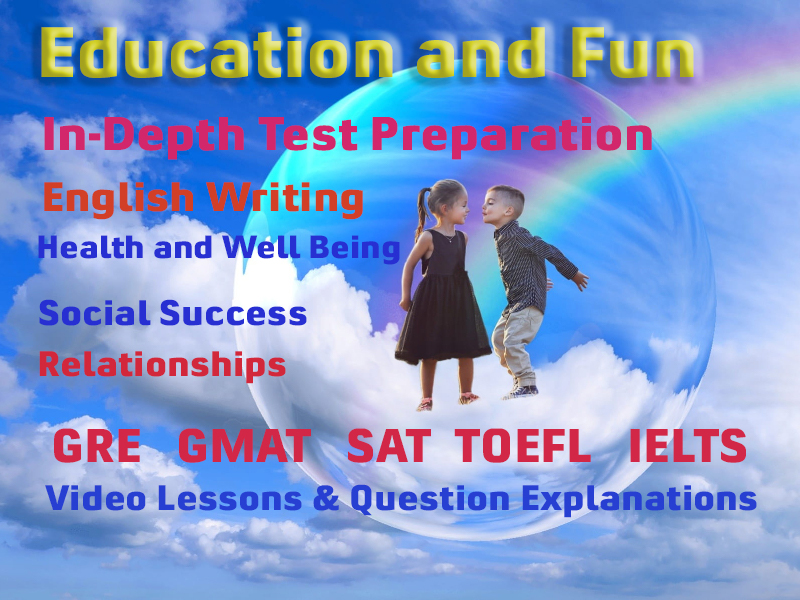# ISSB Missing Figure in Arithmetic Questions

This is an easy test of simple arithmetic where the candidate has to insert missing figures only.

### Example:

Inset the missing figures in the sum given be Each star stands for one figure only:

• 1. Rs. __ 6
• 2. __**6
• 3. 19979 ___ 76

### Simple Arithmetic

You must be proficient in doing simple arithemtic operations of addition, subtraction, multiplication, and division. You can use effectivly, the arithmetic test preparation of this site. You can opt Number system and basic arithmetic from the following modules.

### What is Arithmetic

Arithmetic is a branch of mathematics concerned with the numerical manipulation of numbers using the operations of addition, subtraction, multiplication, division, and the extraction of roots.

General arithmetic principles slowly developed over time from the principle of counting objects. Critical to the advancement of arithmetic was the development of a positional number system and a symbol to represent the quantity zero. All arithmetic knowledge is derived from the primary axioms of addition and multiplication. These axioms describe the rules which apply to all real numbers, including whole numbers, integers, rational, and irrational numbers.

### More Educational and Fun StuffMore In-depth knowledge about what you need. Detailed about test preparation, English Writing, TOEFL, and IELTS.